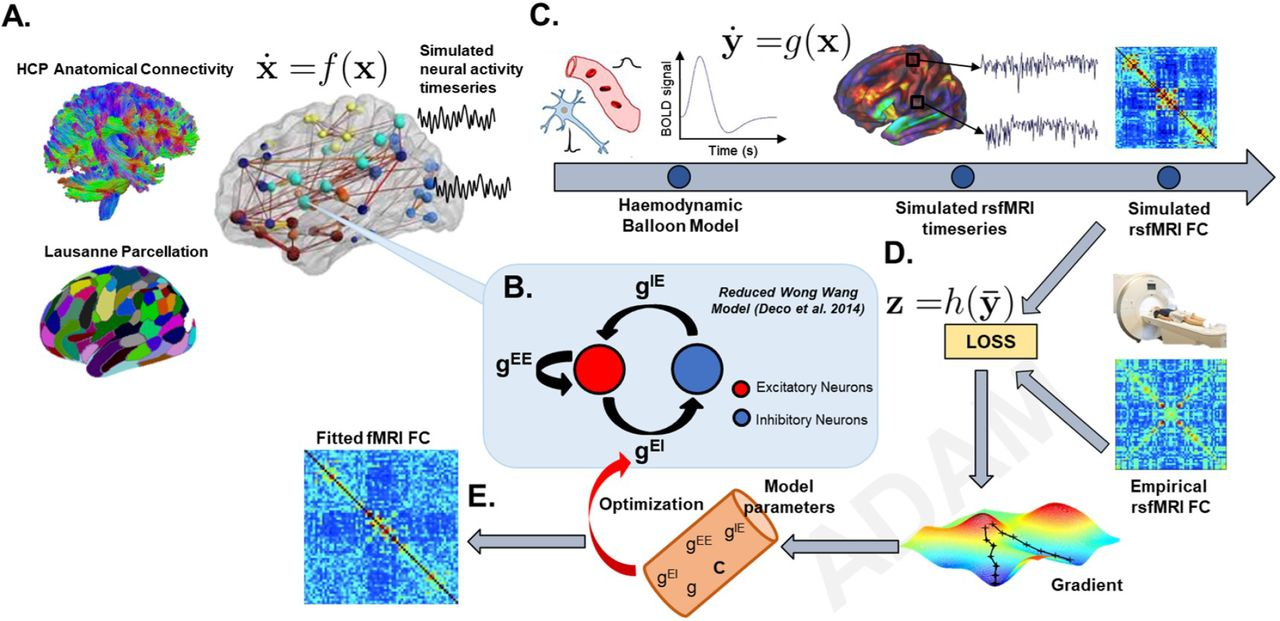# Deep Learning-Based Parameter Estimation for Neurophysiological Models of Neuroimaging Data

May 19, 2022
Griffiths, J.D. and Wang, Z. and Ather, S.H. and Momi, D. and Rich, S. and Diaconescu, A. and McIntosh, A.R. and Shen. K.## Abstract:

Connectome-based neural mass modelling is the emerging computational neuroscience paradigm for simulating large-scale network dynamics observed in whole-brain activity measurements such as fMRI, M/EEG, and related techniques. Estimating physiological parameters by fitting these models to empirical data is challenging however, due to large network sizes, often physiologically detailed fast-timescale system equations, and the need for long (e.g. tens of minutes) simulation runs. Here we introduce a novel approach to connectome-based neural mass model parameter estimation by employing optimization tools developed for deep learning. We cast the system of differential equations representing both neural and haemodynamic activity dynamics as a deep neural network, implemented within a widely used machine learning programming environment (PyTorch). This allows us to use robust industry-standard optimization algorithms, automatic differentiation for computation of gradients, and other useful functionality. The approach is demonstrated using a connectome-based network with nodal dynamics specified by the two-state RWW mean-field neural mass model equations, which we use here as a model of fMRI-measured activity and correlation fluctuations. Additional optimization constraints are explored and prove fruitful, including restricting the model to domains of parameter space near a bifurcation point that yield metastable dynamics. Using these techniques, we first show robust recovery of physiological model parameters in synthetic data and then, as a proof-of-principle, apply the framework to modelling of empirical resting-state fMRI data from the Human Connectome Project database. For resting state activity, the system can be understood as a deep net that receives uncorrelated noise on its input layer, which is transformed into network-wide modelled functional connectivity on its output layer. This is consistent with the prevailing conception in theoretical neuroscience of resting-state functional connectivity patterns as an emergent phenomenon that is driven by (effectively) random activity fluctuations, which are then in turn spatiotemporally filtered by anatomical connectivity and local neural dynamics.